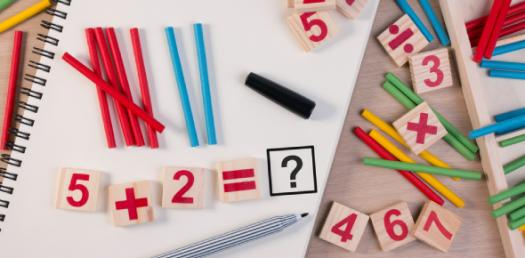# Can You Really Solve This Basic Addition Test? Math Trivia Quiz

48 Questions | Total Attempts: 1035SettingsCan You Really Solve This Basic Addition Test? This trivia math quiz is used to show how easy it would be to solve simple addition problems. A lot of people have a negative relationship with math and it can all change if they seek to have regular practice. How about you take up the quiz and up your skills?

Related Topics
• 1.
5+5=
• 2.
4+6=
• 3.
2+7=
• 4.
1+8=
• 5.
5+2=
• 6.
6+3=
• 7.
4+5=
• 8.
2+8=
• 9.
7+3=
• 10.
5+4=
• 11.
4+3=
• 12.
2+8=
• 13.
6+4=
• 14.
3+7=
• 15.
2+6=
• 16.
1+6=
• 17.
5+3=
• 18.
9+1=
• 19.
5+5=
• 20.
2+3=
• 21.
5+4=
• 22.
4+6=
• 23.
1+9=
• 24.
4+0=
• 25.
1+9=